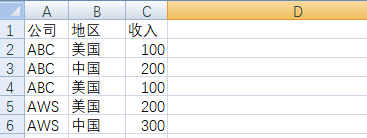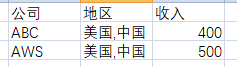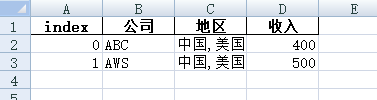# 一、概述

现有一个excel文件examples.xlsx，内容如下：合并去重后，效果如下：那么需求如下：

```公司去重，保留一个

多个地区合并为一行，用逗号隔开

收入进行累计计算```

最后将统计结果，保存到新的excel中。

# 二、演示

完整代码如下：

```# ！/usr/bin/python3
# -*- coding: utf-8 -*-

import pandas as pd

def computer(x):  # 数据计算
return pd.Series({
# 去重
'公司': ','.join(x['公司'].unique()),
'地区':
','.join(list(set((','.join(x['地区']).split(','))))),
'收入': sum(x['收入']),
})

# 读取原始数据excel
# 使用groupby进行分组
res = _df[['公司', '地区', '收入']].groupby(['公司'], as_index=False).apply(computer).reset_index()
# 保存到新的excel中